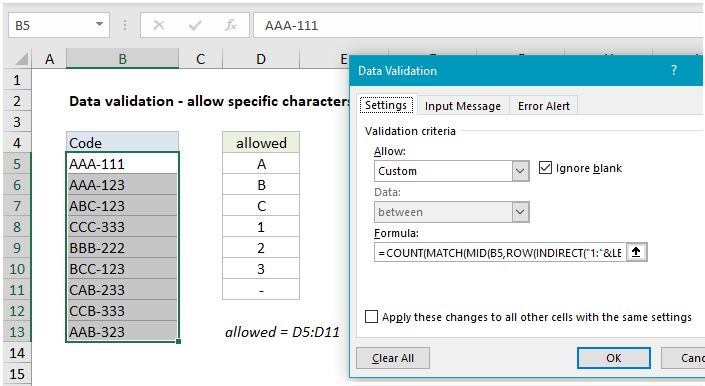## Excel Office

Excel How Tos, Tutorials, Tips & Tricks, Shortcuts

# Set criteria to accept specific characters only

To use data validation to allow a list of specific characters only, you can use a rather complicated array formula based on the COUNT, MATCH, and LEN functions.

## Formula

```=COUNT(MATCH(MID(A1,ROW(INDIRECT
("1:"&LEN(A1))),1),allowed&"",0))=LEN(A1)```

##Explanation

In the example shown, data validation is applied with this formula:

```=COUNT(MATCH(MID(B5,ROW(INDIRECT
("1:"&LEN(B5))),1),allowed&"",0))=LEN(B5)```

where “allowed” is the named range D5:D11.

### How this formula works

Working from the inside out, the MID function is used to generate an array from text entered in B5 with this snippet:

`MID(B5,ROW(INDIRECT("1:"&LEN(B5))),1)`

explained in detail here. The result is an array like this:

`{"A";"A";"A";"-";"1";"1";"1"}`

which goes into MATCH as the lookup value. For lookup array, we use the named range “allowed”, concatenated to an empty string (“”):

`allowed&""`

The concatenation converts any numbers to strings, so that we are matching apples-to-apples. The result is an array like this:

`{"A";"B";"C";"1";"2";"3";"-"}`

The last argument in MATCH, match_type is set to zero to force an exact match. Because we give MATCH multiple lookup values, we get back an array with multiple results:

`{1;1;1;7;4;4;4}`

Each number in this array represents a match. In the event a match isn’t found for a character, the array will contain a #N/A error.

Also See:   Remove last characters from right in a cell in Excel

Finally, the COUNT function is used count the numbers in the result array, which is compared to a count of all characters in the cell calculated with the LEN function. When MATCH finds a match for all characters, the counts are equal, the formula returns TRUE, and data validation succeeds. If MATCH doesn’t find a match any character, it returns #N/A instead of a number. In that case, the counts don’t match and data validation fails.

Also See:   Get work hours between dates custom schedule in Excel